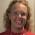A problem with a chord: find the radius

Today I had the opportunity to solve a real math problem involving a circle and a chord of known length in it. I had to find the radius. It wasn't a textbook problem or a puzzle on some website, but a math problem I needed to solve for my own needs.

For a tiny while I thought I could find the answer online, but I didn't, so I'm writing it out in case someone else needs it -- they should be able to find this solution by searching the Internet.

I wanted to make a kind of "moon-sliver shapes" in CorelDraw, to use as watermarks in my new books. I have the height and the width of the "sliver".

Here's the problem mathematically:
I have a chord of a circle, 17 mm in length in my example, and the other distance marked in the image is 5 mm. I need to find the radius of the circle, AND the angle measure of the arc of the circle that makes the sliver's rounded part.At first, like I said, I searched around if there was some theorem or formula that would tell me what I needed directly. I didn't find any, but I did realize that I can use this theorem to solve my problem:

If two chords intersect, the product of the segments of one chord equals the product of the segments of the other chord (see proof).

My problem looks like this:My chord intersects the diameter of the circle, which is a chord too. The two parts of the first cord are 8.5 and 8.5, and the two parts of the other are 5 and d − 5. Thus I get the equation

5(d − 5) = 8.52

From this it is quick to solve that d = 19.45. Then, the radius is of course half that, or 9.725.

On to the second part of my problem: to find the angle measure.

In this picture, I have a right triangle. I can therefore find the unknown angle α by using simple trigonometry, in this case the tangent.The length 4.725 comes from the fact that the radius is 9.725, and then I subtract 5 from that.

The equation is tan α = 8.5/4.725, from which α ≈ 60.931°. The actual angle I want is double that, or about 121.862°.

I had to repeat this calculation several times for slivers of different "heights." (Or, actually I let Excel calculate the rest. Oh, how I love Excel!)

Here's one of the final pictures I made for my book:Saima Rizvi said…
How did u get the equation
5(d-5) = 8.5^2Maria Miller said…
It comes from using the theorem that I quote, "If two chords intersect, the product of the segments of one chord equals the product of the segments of the other chord."

5 and d-5 are the segments of the one cord, and 8.5 and 8.5 are the segments of the other.ron said…
Looks good.

See math has its practicall applications

:)Trung said…
Alternately, you could set up a right triangle. One leg is 8.5 mm, the other leg is R - 5, and the hypotenuse is R. Use the Pythagorean Theorem and solve the quadratic equation. Cheers!David Chandler said…
That's the theorem used by highway crash investigators in measuring the radius of curvature for a curve on a road. The maximum speed depends on the radius of curvature.Anonymous said…
Where m is equal to the distance from the midpoint of the segment to to circle perpendicularly or the "height" of the segment and C the length of the segment within the circle